# CBSE 12th boards 2018: Sample paper for physics, test your preparation

PUBLISH DATE 6th February 2018CBSE class 12 boards 2018: The board exams for both class 10 and 12 are just one month away and students must be busy revising and polishing their last month preparation. The Central Board of Secondary Education (CBSE) will conduct class 12 exams from March 5 till April 12. A score of at least 33 per cent in their internals and practicals is required to pass the subject and at least 33 per cent in the board theory examination to clear the subject as a whole. Check out the sample paper for physics and try attempting the same. This will help you in time management and will also give you a rough idea regarding your level of preparation.

Section A: contains five questions of one mark each, Section B contains five questions of two marks each, Section C contains twelve questions of three marks each, Section D contains one value based question of four marks and Section E contains three questions of five marks each.

There is no overall choice. However, an internal choice has been provided in one question of two marks, one question of three marks and all the three questions of five marks weightage. You must attempt only one of the choices in such questions.

You may use the following values of physical constants wherever necessary.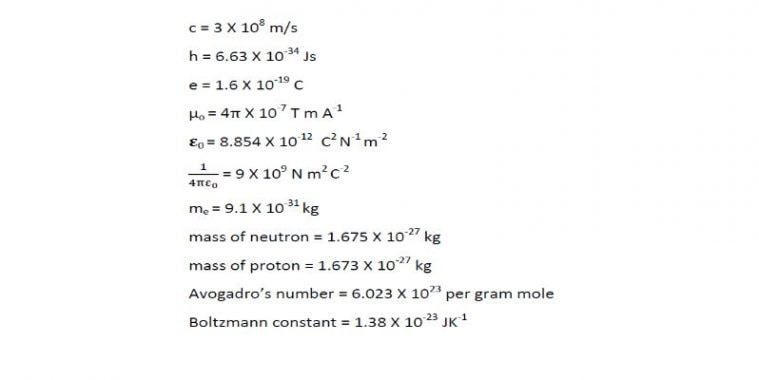Section – A

1. What type of wave-front will emerge from a (i) point source, and (ii) distant light source ?
2. You are asked to measure the emf of a cell. Which instruments will you use, a high resistance voltmeter or a potentiometer and why?
3. The focal length of an equi- convex lens is equal to the radius of curvature of either face. What is the refractive index of the material of the lens?
4. What is the angle between the direction of electric dipole moment and direction of electric field at any point on (i) axial line and (ii) equatorial line, of the dipole?
5. What is the ratio of radii of second permitted stationary orbit to the fourth permitted stationary orbit of hydrogen or hydrogen like atoms?

Section – B

6. (a) Draw a graph between angle of deviation and angle of incidence for a prism.
(b) Calculate the angle of minimum deviation for an equilateral triangular prism of refractive index.

7.  The time varying electric and magnetic field will produce an electromagnetic wave. The electric field of an        electromagnetic wave is given by
Ex = E o Sin (kz – ωt)

a) Write the direction of propagation of electromagnetic wave.
b) The magnetic field of a radio wave can be represented as B= (40 X10 -6 T) Sin (0.4×10 3 x+2.5×10 10 t).
What is the amplitude of electric field vector?

8. A voltage source is connected to an electrical component X as shown in figure. If phase angle between current and voltage is 90 for X then,

a) Identify the device X. Write down an expression for the current through X.
b) Draw the phasor diagram for the circuit element X.

9. Explain the term side band frequency and Band width of signal. What will be the Band width of signal used for music and T.V. broadcast?

Or

Draw the block diagram of basic element of communication system.

10. LEDs that can emit red, yellow, orange, etc commercially available.
a) How these colours are obtained in a LED ?
b) As the forward bias current increases, intensity of light emitted by LED increases and reaches a maximum. Further increase in forward current decreases the light intensity. Why?

Section – C

11. a) “Electric field lines are always parallel to equipotential surfaces”. Correct the statement if there is any mistake.
b) Draw the equipotential for a single positive point charge.
c) A point charge +q is placed at the centre of a sphere of radius R. Another point charge +q is taken from a point            A to another diametrically opposite point B on the surface of the sphere. Calculate the work done for this.

12. An a.c. source of 110V with variable frequency is connected to a series LCR circuit. (Given L = 0.38 H, C = 27 μ F and R = 48 Ω). At a particular frequency ‘ω’ the voltage across the capacitor and inductor are found to be equal.
a) Find the current through the circuit at frequency ω.
b) How does the circuit current change if the plates of the capacitor are brought closer?

13. Using Rutherford model of the atom, derive the expression for the total energy of the electron in hydrogen atom. What is the significance of total negative energy possessed by the electron?

14. a) Derive the expression for force per unit length between two long straight parallel current carrying conductors.
b) Increasing the current sensitivity of a galvanometer may not necessarily increase its voltage sensitivity. Why?

15. a) What happens to the value of L of the coil when the no of turns is doubled keeping all other parameters constant?
b) An induced emf of 4V is generated when the current in the circuit changes from 3 A to 4A in one millisecond. What is the self-inductance of the coil?
c) Write two applications of eddy currents.

16. State Gauss theorem. Use Gauss theorem to obtain the expression for electric field due to uniformly charged long straight wire. Sketch the graphical variation of electric field with distance r from wire.

17. In the following diagram, an object ‘O’ is placed 15 cm in front of a convex lens L 1 of focal length 20 cm and the final image is formed at ‘I’ at a distance of 80 cm from the second lens L 2 . Find the focal length of the lens L 2 .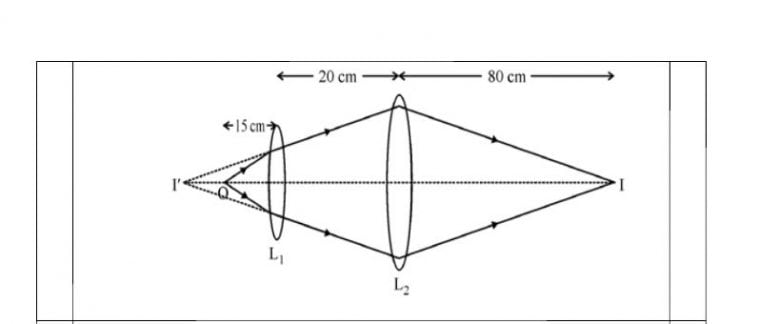18. A long narrow slit is illuminated by blue light and the diffraction pattern is obtained on a white screen.
a) How the width of fringes change as the distance of the slit from the centre of the screen increases?
b) What happens to the width of pattern, if yellow light is used instead of blue light?
c) In a double slit experiment, the slits are separated by 0.03 cm and the screen is placed 1.5 m away. The distance between the central fringe and the fourth bright fringe is 1 cm. Determine the wavelength of light used in the experiment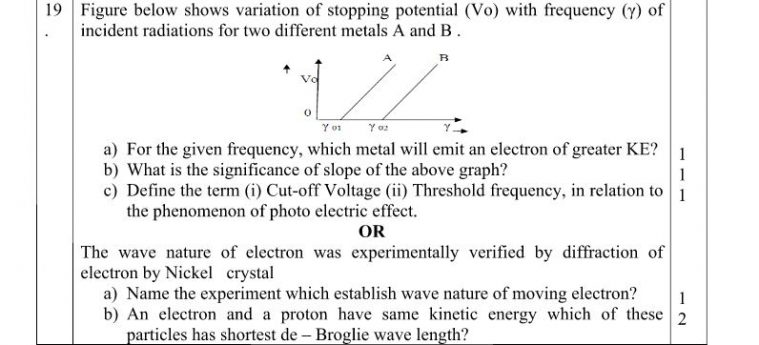20. A student was asked to compare the resistance of two conductors using a meter bridge apparatus.
a) What is the working principle of Meter Bridge?
b) Draw the necessary circuit diagram for the experiment.
c) Why this arrangement is not suitable for measuring very small resistances?

21. Nuclear radius depends on the mass number of the element.
a) Write down the expression for nuclear radius.
b) A radioactive isotope has a half-life of T years. How long will it take the activity to reduce 3.125% of its original        value?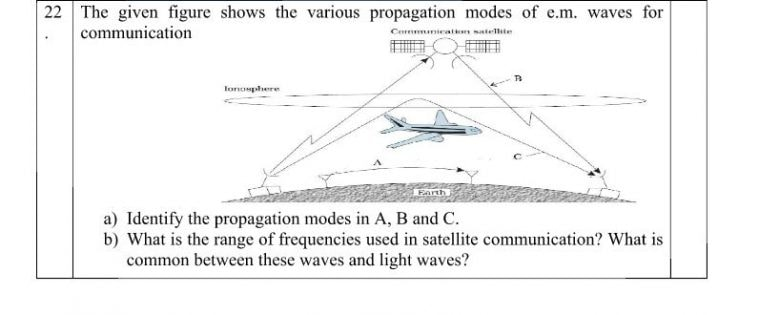Section – D (Value Based Question)

23. Supraja was doing an experiment (Comparison of emfs ) using potentiometer in Physics lab. She could not take the readings because the galvanometer showed same side deflection. She checked the circuit and the connections were correct. Her friend Manasa who was doing her experiment nearby came to help Supraja. Manasa increased the voltage of the eliminator (by turning the knob) supplying current to the potentiometer. Supraja tried the experiment again and got the readings. She thanked Manasa for her help.

a) What are the values displayed by both Supraja and Manasa?
b) State any two reasons why the galvanometer showed same side deflection.

Section – E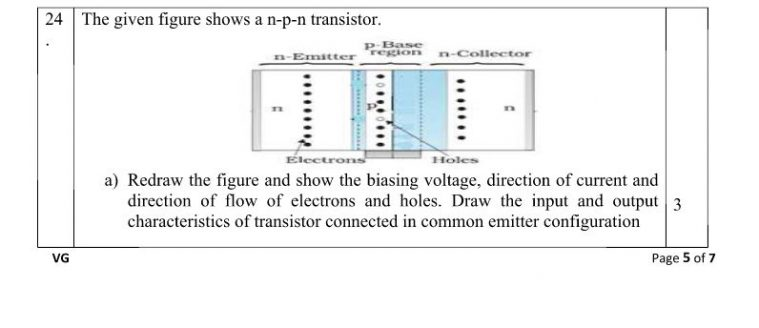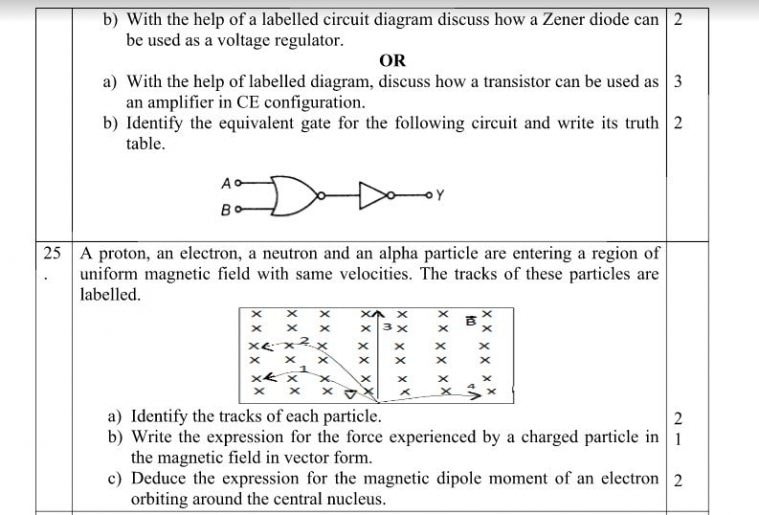26. According to a principle, at a particular point in a medium, the resultant displacement produced by a number of waves is the vector sum of the displacements produced by each of the waves.
(a) Derive an expression for the fringe width in Young’s double slit experiment.
(b) Find the ratio of intensities at two points P and Q on a screen in Young’s double slit experiment when waves from sources S 1 and S 2 have phase difference of (i) 0 0 and (ii) π/2 respectively.

OR

a) Draw a labelled diagram of Astronomical telescope when image is formed at least distance of distinct vision.
b) A compound microscope has a magnification of 30. The focal length of its eyepiece is 5 cm. assuming the final image to be formed at the least distance of distinct vision, calculate the magnification produced by the objective.
c) How the resolving power of Compound microscope change with the change in the refractive index of the medium between object and objective lens?

--------------------------------------------------------------------------------------------------------------------------------------------------------

Good NEWS 12th Science

Hello Students,

VidyarthiMitra.Org & Icon Science Hub, Pune Best Coaching Institute for (11th + 12th, IIT-JEE / NEET) are  LIVE on Facebook, for your ensuing Board Exams [HSC/CBSE, (PCMB)] 2018. Tips and Tricks on: How to Score Maximum; How to & What to Study Now ??

By Our Subject Experts, on 9th Feb 2018 at 6 : 00 pm. Please send your doubts and queries on WhatsApp, so we can specifically clear them. Register your presence on WhatsApp 7720025900 or on Facebook

--------------------------------------------------------------------------------------------------------------------------------------------------------

Also Read: MHT CET       NDA    NEET UG   JEE Main exam.    JEE Advanced

Registrations for MOCK EXAMS Commencing Soon click to Notications

--------------------------------------------------------------------------------------------------------------------------------------------------------
Get free Educational & jobs alert on WhatsApp OR Telegram(https://t.me/VidyarthiMitra) Save this mobile number (77200 25900) on your phone as VidyarthiMitra.org and send WHATSAPP message (Your Name, City & Interest)

--------------------------------------------------------------------------------------------------------------------------------------------------------
'विद्यार्थी मित्र' जॉईन करा आणि मिळवा न्यूज, नोकरी, शासकीय व निमशासकीय नोकऱ्यांच्या जाहिराती व माहिती अगदी विनामूल्य ते ही आपल्या व्हॉटस्अॅपवर किंवा* *टेलेग्राम (https://t.me/VidyarthiMitra) हा मोबाईल नं. सेव्ह करून आपले <नाव> <शहराचे नाव> <नोकरी> ७७२०० २५९०० मेसेज पाठवा

--------------------------------------------------------------------------------------------------------------------------------------------------------

#### Related News